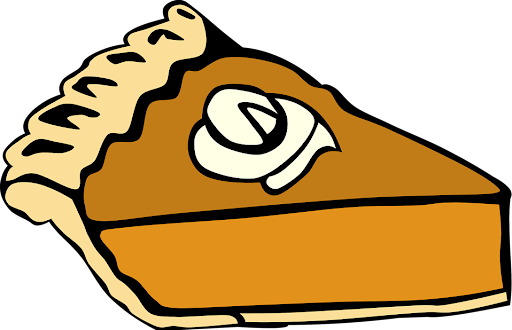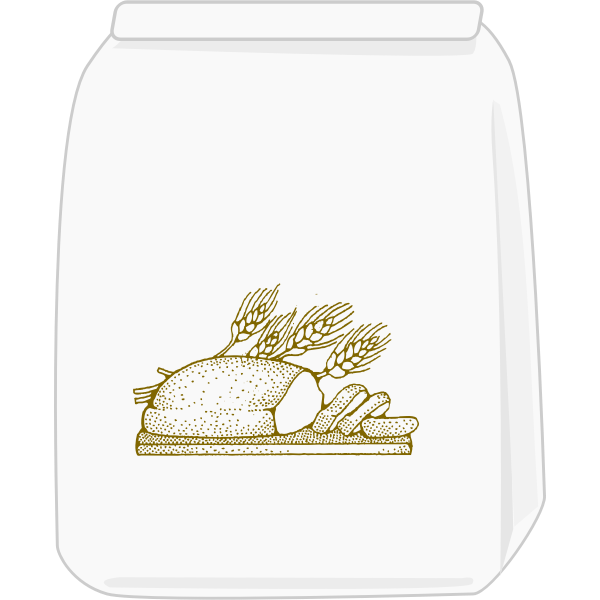# Math help from the Learning Centre

This guide provides useful resources for a wide variety of math topics. It is targeted at students enrolled in a math course or any other Centennial course that requires math knowledge and skills.

## Introduction

There are two situations that may occur in dealing with kitchen ratios: the total amount to be made can be known or unknown.

Example for Known situation:

A recipe asking for 10 ounces of pie dough. In this case, you know the total amount you need to make with the pie dough ratio.

Example for Unknown situation:

You have 10 ounces of flour and want to use it to make pie dough. The total amount to be made is unknown, but you do know the amount of one of the ingredients.## Known Total Amount

There are four steps for determining ingredient quantities when you know the total amount that needs to be made:

1. Determine the total quantity to be made.

2. Find the total number of parts in the ratio.

3. Find the amount per part by dividing the total quantity to be made by the total number of parts.

4. Find the amount of each ingredient by multiplying each ingredient by the amount per part.

Example

You need 12 pounds of pie dough. How many pounds of each ingredient should you use? The ratio of ingredients to make pie dough is as follows:

• 3 parts flour
• 2 parts oil
• 1 part water

Solution

In this case, we know the total amount of pie dough to be made (12 pounds). Using the given information, the total number of parts in the ratio is calculated as:

3 parts flour + 2 parts oil + 1 part water = 6 parts total

We now find the amount per part by dividing the total quantity to be made by the number of parts total:

12 pounds of pie dough $$\div$$ 6 parts total = 2 pounds per part

Finally, we multiply the parts per ingredient by the amount per part to find the total amount of each ingredient we need:

3 parts flour x 2 pounds per part = 6 pounds of flour

2 parts oil x 2 pounds per part = 4 pounds of oil

1 part flour x 2 pounds per part = 2 pounds of water

## Unknown Total Amount

There are two steps for determining ingredient quantities when the total to be made is unknown:

1. Find the amount per part by dividing the amount that you know by the number of parts it represents.

2. Multiply the amount per part by the number of parts for each of the remaining ingredients.

Example

You have 6 pounds of flour to make pie dough. How many pounds of oil and how many pounds of water should you add to the flour? The ratio of ingredients to make pie dough is as follows:

• 3 parts flour
• 2 parts oil
• 1 part water

Solution

In this case, we do not know the total amount of pie dough that will be made. So, we divide the amount of the ingredient we know (flour) by the number of parts it represents:

6 pounds of flour $$\div$$ 3 parts = 2 pounds per part

Finally, we can multiply the amount per part by the number of parts for each of the ingredients we want to determine amounts for:

Oil: 2 parts x 2 pounds per part = 4 pounds of oil

Water: 1 part x 2 pounds per part = 2 pounds of water